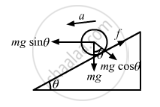Advertisement Remove all ads

# A Hollow Sphere is Released from the Top of an Inclined Plane of Inclination θ. (A) What Should Be the Minimum Coefficient of Friction Between the Sphere and the Plane to Prevent Sliding? - Physics

Sum

A hollow sphere is released from the top of an inclined plane of inclination θ. (a) What should be the minimum coefficient of friction between the sphere and the plane to prevent sliding? (b) Find the kinetic energy of the ball as it moves down a length l on the incline if the friction coefficient is half the value calculated in part (a).

Advertisement Remove all ads

#### Solution

It is given that a hollow sphere is released from the top of an inclined plane of inclination θ.

(a) To prevent sliding, the body will make only perfect rolling. In this condition, we have

$mgl \sin \theta - f = ma............(1)$

$f \times R = \left( \frac{2}{3} \right) m R^2 \times \left( \frac{a}{R} \right)$

$\Rightarrow f = \frac{2}{3} ma...........(2)$

On putting this value in the equation (1), we get

$mg \sin \theta - \frac{2}{3} ma = ma$

$\Rightarrow a = \frac{3}{5} g \sin \theta$

From equation (1), we have

$mg \sin \theta - f = \frac{3}{5} mg \sin \theta$

$\Rightarrow f = \frac{2}{5} mg \sin \theta$

$\Rightarrow \mu mg \cos \theta = \frac{2}{5} mg \sin\theta$

$\Rightarrow \mu = \frac{2}{5} \tan \theta$

(b)

$\left( \frac{1}{5} \right) \tan \theta \left( mg \cos \theta \right) R = \frac{2}{3} m R^2 \alpha$

$\Rightarrow \alpha = \frac{3}{10} \left( \frac{g \sin \theta}{R} \right)$

$a_c = g \sin \theta - \left( \frac{g}{5} \right) \sin \theta$

$= \left( \frac{4}{5} \right) g \sin \theta$

$\Rightarrow t^2 = \frac{2l}{a_c}$

$= 2l \left( 4g\frac{\sin \theta}{5} \right) \left( \frac{5}{2g \sin \theta} \right)$

$\therefore \omega = at$

$K . E . = \frac{1}{2} m \nu^2 + \frac{1}{2} I \omega^2$

$= \frac{1}{2} m \left( 2al \right) + \frac{1}{2} l \left( a^2 t^2 \right)$

$= \frac{1}{2} m \left( 4g \frac{\sin \theta}{5} \right) \times 2 \times l + \frac{1}{2} \times \frac{2}{3} m R^2 \times \frac{9}{100}$

$= \left( \frac{\sin^2 \theta}{R} \right) \times \left( \frac{5L}{2g \sin \theta} \right)$

$= 4 mgl \frac{\sin \theta}{5} + 3 mgl \frac{\sin \theta}{40}$

$= \frac{7}{8} mgl \sin \theta$Is there an error in this question or solution?
Advertisement Remove all ads

#### APPEARS IN

HC Verma Class 11, 12 Concepts of Physics 1
Chapter 10 Rotational Mechanics
Q 76 | Page 200
Advertisement Remove all ads

#### Video TutorialsVIEW ALL 

Advertisement Remove all ads
Share
Notifications

View all notifications

Forgot password?